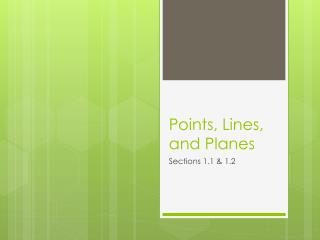DownloadDownload PresentationPoints, Lines, and Planes

# Points, Lines, and Planes

Télécharger la présentation## Points, Lines, and Planes

- - - - - - - - - - - - - - - - - - - - - - - - - - - E N D - - - - - - - - - - - - - - - - - - - - - - - - - - -
##### Presentation Transcript

1. Points, Lines, and Planes Sections 1.1 & 1.2

2. Definition of a Point A point has no dimension. It is represented by a dot. A point is symbolized using an upper-case letter.

3. Definition: Line A line has one dimension. (infinite length) A line is named using any two points on the line with a two sided arrow above them like this: . It can also be named by using a lower-case cursive letter. Through any two points, there is exactly one line.

4. Definition: Collinear points points that lie on the same line.

5. Definition: Between If a point is between two other points then that means it must also be collinear with the other two points.

6. Definition: Plane A plane has two dimensions. It is represented by a shape that looks like a floor or a wall, but it extends infinitely in length and width. Any three non-collinear points can define a plane. A plane is named using the word plane with 3 non-collinear points or with an upper-case cursive letter.

7. Definition: Coplanar: Coplanar points are points that lie (or could lie) in the same plane.

8. Definition: Line Segment: The line segment consists of two endpoints and all the points between them. A line segment is named using both endpoints with a line above them like this: . and refer to the same line segment.

9. Definition: Ray The ray consists of an endpoint and all points on a line in the opposite direction. A ray is named using its endpoint first and then any other point on the ray with a ray symbol pointing to the right above them like this: . and do not refer to the same ray.

10. Definition: Opposite Rays: If point C lies on line AB between A and B, then ray CA and ray CB are opposite rays. Two opposite rays make a line.

11. Definition: Intersection: The intersection of two or more figures is the set of points the figures have in common. The intersection of 2 different lines is a point. The intersection of 2 different planes is a line.

12. Definition: Distance: The distance between points A and B, also known as the length of line segment AB is the absolute value of the difference of the coordinates of A and B. In other words, distance is many units apart the points lie. The distance from A to B, or the length of Is written as AB. (No symbol above).

13. Definitions: Postulate: A rule that is accepted without proof. Theorem: A rule that can be proven.

14. The Ruler Postulate: The points on a line can be matched one to one with the real numbers.

15. Segment Addition Postulate: If B is between A and C, then AB + BC = AC. If AB + BC = AC, then B is between A and C.

16. Definition: Congruent Segments: Line segments that equal (=)length are called congruent (segments. To show that two segments are congruent in a drawing we use tick marks. A B C D

17. PRACTICE: A1 • p.5: 1-12, 17-22 • p.12: 6-10(even), 16-30(even)